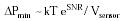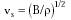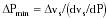Nanomedicine, Volume I: Basic Capabilities

Robert A. Freitas Jr., Nanomedicine, Volume I: Basic Capabilities, Landes Bioscience, Georgetown, TX, 1999

4.5.1 Minimum Detectable Pressure

A change in the ambient pressure in a fluid environment (DP) may be determined by measuring the change in volume (DV) of a fixed quantity of gas at constant temperature, relative to a known sensor volume at a known reference pressure. (Thermal sensors elsewhere in the nanorobot provide readings by which corrections can be made for temperature variations.) If the sensor volume consists of a cylinder of fixed cross-sectional area, the change in volume is converted into a linear displacement of a piston located at the interface between gas and fluid environment. The change in Gibbs free energy10 is DG = DP DV, while thermal noise ~kT. Pressure sensor signal/noise ratio SNR ~ ln (DP DV / kT), so at the minimum DG the minimum detectable pressure DPmin is measured at the maximum possible volume change, or Vsensor (the sensor volume), given approximately by{Eqn. 4.29}

using the classical ideal gas formulation. At SNR = 2, a (22 nm)3 = 104 nm3 sensor (~5 x 105 atoms or ~10-20 kg for a cube with 1 nm thick walls) can detect a minimum pressure change DPmin ~ 0.03 atm; a (680 nm)3 = 0.3 micron3 sensor (~3 x 109 atoms, ~6 x 10-17 kg) detects DPmin ~ 10-6 atm, probably near the practical limit for mobile in vivo medical nanodevices. Note that DPmin scales approximately as the inverse cube of mean sensor dimension, and immunity to thermal noise scales exponentially with sensor volume. Current silicon micromachined pressure sensors are ~1 mm3 with 10-3 atm sensitivity,456,457 a factor of ~1012 poorer sensitivity than the theoretical minimum for their size. (A field-effect chemical sensor with an active sensing volume of 0.1 mm3 that measures pressure indirectly has detected a 10-11 atm step in hydrogen gas with tmeas ~ 3 sec,458 only a factor of 1000 above the theoretical minimum for a sensor of that size.) The human ear can detect pressure waves as small as 2 x 10-10 atm.

A less sensitive approach relies on the observation that a change in pressure P alters both density and bulk modulus of a compressed fluid, variations that may be detected by measuring the change in the speed of sound vs in the working fluid, given by{Eqn. 4.30}

where B is bulk modulus of the fluid and r is fluid density. (dB/dP)/B may be up to several times larger than (dr/dP)/r for molecular fluids, but there is some (dvs/dP)/vs for each fluid. Differentiating Eqn. 4.30 with respect to P and using dr/dP = r/B, then dvs/dP = (dB/dP - 1) / 2 r1/2 B1/2. For water at 1 atm, dB/dP ~ 8.4 atm/atm,567 r ~1000 kg/m3 and B = 22,000 atm, giving dvs/dP ~ 0.25 m/sec-atm. If Dvs is the measured change in acoustic speed, then:{Eqn. 4.31}

Velocity can be measured to ~0.1 mm/sec accuracy using a micron-scale velocity sensor and tmeas ~ 1 sec (Section 4.3.2), so a minimum Dvs ~ 0.1 mm/sec implies DPmin ~ 0.0004 atm.

Last updated on 17 February 2003